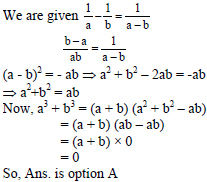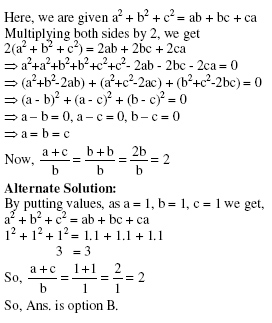# Sample Aptitude Questions of SAP

1. The average score of a cricketer for 13 matches is 42 runs. If his average score for the first 5 matches is 54, then what was his average score (in runs) for last 8 matches?
1. 37
2. 39
3. 34.5
4. 33.5

Total Score = Average * Number of matches
Total score of 13 matches = 13 × 42 = 546
Total score of first 5 matches = 5 × 54 = 270
Therefore, total score of last 8 matches = 546 - 270 = 276
Average = 276/8 = 34.5
Hence the answer is option C
2. If 1/a - 1/b = 1/a-b, then the value of a3+b3is
1. 0
2. -1
3. 1
4. 21. If a2+b2+c2 = ab+bc+ca , then (a+c)/b is equal to
1. 1
2. 2
3. 3
4. 42. If the graphs of the equations x + y = 0 and 5y + 7x = 24 intersect at (m, n), then the value of m +n is
1. 2
2. 1
3. 0
4. -1

By solving the given two equations, we get the intersection point (12, - 12).
So, m = 12, n = -12
Hence, m + n = 12 – 12 = 0 So, ans. is option C.
3. A function f(x) is defined as f(x) = f(x – 2) - x(x + 2) for all the integer values of x and f(1) + f(4) = 0. What is the value of f(1) + f(2) + f(3) + f(4) + f(5) + f(6)?
1. 0
2. 89
3. -89
4. None of these

Let S = f(1) + f(2) + f(3) + f(4) + f(5) + f(6)
As f(1) + f(4) = 0, therefore S = f(2) + f(3) + f(5) + f(6) ------ (1)
f(2) = f(0) - 8
f(3) = f(1) - 15
f(4) = f(2) - 24 = f(0) - 32
f(5) = f(3) - 35 = f(1) - 50
f(6) = f(4) - 48 = f(0) - 80
Put the above values in equation (1), we get
S = f(0) - 8 + f(1) - 15 + f(1) - 50 + f(0) - 80
S = 2(f(0) + f(1)) - 153 ------ (2)
As we already know f(1) + f(4) = 0 ⇒f(1) + f(0) - 32 = 0 ⇒f(1) + f(0) = 32
Putting this value in equation 2, we get S = 2(32) - 153 = -89
So, Ans is option C.
4. What annual payment will discharge a debt of Rs. 6,450 due in 4 years at 5% per annum simple interest?
1. 1400
2. 1500
3. 1550
4. 1600
5. None of these

Let the annual installment be rs. x
therefore (x + x*3*5/100)+(x + x*2*5/100)
+(x + x*1*5/100)+x =6450
=>115x/100+110x/100+105x/100+x=6450
=>115x+110x+105x+100x=6450*100
=>430x=6450*100
x=6450*100/430=rs.1500
5. The average of the first 100 positive integers is
1. 100
2. 51
3. 50.5
4. 49.5
5. 49

n(n+1)/2
1+2+3+....+n
therefore average of these numbers=n+1/2
therefore required average
100+1/2=50.5
6. In a family, the average age of a father and a mother is 35 years. The average age of the father, mother and their only son is 27 years. What is the age of the son?
1. 12 years
2. 11 years
3. 10.5 years
4. 10 years
5. None of these

Father+Mother=2*35=70 years
Father+Mother+Son=27*3=81 years
therefore Son's age=81-70=11 years
DIRECTIONS for the question 9 and 10 : Solve the following question and mark the most appropriate option
7. The length and breadth of a rectangle are increased by 20% and 40% respectively. What is the percentage increase in its area?
1. 60%
2. 68%
3. 78%